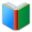# List 1 for 2nd Grade MATH

Elementary School Words: List 1 for 2nd Grade MATH, all double sided cards of the list are in one page. You can download and print the worksheet from browser directly.
 Actions upon current list

 All lists of current grdae
Sight Words:

Arts Words:

Social Words:

Math Words:

Science Words:

Literature Words:The Real PrincessThe Old HouseThe Frog PrinceBriar RoseRapunzelThe Robber BridegroomKing Grisly-BeardLittle Red CapThe Sweetheart Roland
 Worksheet - Elementary School Words: List 1 for 2nd Grade MATH

 Print: List 1 for 2nd Grade MATH

Exam Word - https://www.examword.com/

Elementary School Words: List 1 for 2nd Grade MATH

 centimeter a metric unit of length equal to one hundredth of a meter
 congruent possessing congruity; suitable; agreeing; corresponding
 equivalent being essentially equal to something
 even occurring at fixed intervals; not odd; capable of division by two without a remainder
 expanded notation showing place value by multiplying each digit in a number by the appropriate power of 10
 Fahrenheit of or relating to a temperature scale proposed by the inventor of the mercury thermometer
 feet a unit of length in the U.S. Customary and British Imperial systems equal to 12 inches
 fourth one of four equal parts
 fraction segment; fragment; a small proportion of
 growing relating to or suitable for growth
 half one of two divisions; consisting of one of two equivalent parts in value or quantity
 hundreds numerical value equal to 100
 inch a unit of measurement for advertising space; a unit of length equal to one twelfth of a foot
 meter the basic unit of length adopted under
 notation technical system of symbols used to represent special things; comment or instruction
 odd not easily explained; beyond from the usual or expected; not divisible by two
 operation a process or action, such as addition, substitution, transposition, or differentiation, performed in a specified sequence and in accordance with specific rules
 pictograph a graphic character used in picture writing
 probability a measure of how likely it is that some event will occur; the quality of being probable
 repeating the act of doing or performing again
 ruler measuring stick with a straight edge that is used for drawing straight lines and measuring lengths
 standard criteria; basis for comparison
 standard-unit units commonly encountered for a particular quantity
 symmetric having similarity in size, shape, and relative position of corresponding parts
 third following the second position in an ordering or series; one of three equal parts of a divisible whole
 yard rod; stick; branch; long piece of timber; measure of length, equaling three feet